# Average speed

When the bus stops at bus stops driving average speed is 45 km/h. If it did not stop it drive at speed 54 km/h. How many minutes of every hour it spend at stops?

Result

t =  10 min

#### Solution:

45*1 = 54*(1-t/60)

54t = 540

t = 10

Calculated by our simple equation calculator.

Leave us a comment of example and its solution (i.e. if it is still somewhat unclear...):Be the first to comment!#### To solve this verbal math problem are needed these knowledge from mathematics:

Need help calculate sum, simplify or multiply fractions? Try our fraction calculator. Do you have a linear equation or system of equations and looking for its solution? Or do you have quadratic equation?

## Next similar examples:

1. Car driver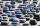The car driver is in town A and is scheduled to be in town B at a specified hour. If it travels at an average speed of 50km/h, it will arrive in city B 30 minutes later. But if he travels at an average speed of 70km/h, he would arrive half an hour earlier
2. Klara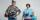Klara and Jitka went on a hiking trip at 13 o'clock at speed 5km/h. At 14 o'clock, Tomas ride on the bike at an average speed of 28 km/h. How many hours and at what distance from the beginning of the road Tomáš caught the two girls?
3. Two planes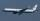Two planes flying from airports A and B, 420 km distant against each other. Plane from A took off 15 minutes later and flies at an average speed of 40 km/h higher than the plane from B. Determine the average speed of this two aircraft if you know that it
4. Two shipsThe distance from A to B is 300km. At 7 am started from A to B a ferry with speed higher by 20 km/h than a ship that leaves at 8 o'clock from B to A. Both met at 10h 24min. Determine how far they will meet from A and when they reach the destination.
5. The cruise ship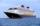The cruise ship has a speed of 12 km / h at a calm surface. When we sail 45 km along the river and 45 km back, it took us exactly 8 hours. Which (constant) speed of flow of the river?
6. Walkers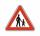From points A and B simultaneously started against each other two walkers. After meeting both continue to B. Second walker came to B 2 hours before the first walker. It's speed is 2.7 times of speed of the first pedestrian. How many hours went pedestria
7. Train speedTwo guns were fired from the same place at an interval of 10 minutes and 30 seconds, but a person in a train approaching the place hears second shot 10 minutes after the first. The speed of the train (in km/hr), supposing that sound travels at 340 m/s is:
8. Aircraft nose downHow long will fall airliner from a height of 10000 m at speed 1,000 km/h?
9. Two cars 2Two cars started from two positions 87 km distant at the same time in opposite directions at speeds 81 km/h and 75 km/h. What was the distance between them after 2 hours 50 minutes of driving.
10. Cyclist vs car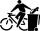Cyclist rode out of the city at 18 km/h. 1 hour 30 minutes behind him started car and caught up with the cyclist in 50 minutes. How fast was driving the car? Where (what kilometer) from the city car overtook a cyclist?
11. Boys and girlsThere are 28 girls in the hall. 5/7 of all children are boys. How many children and how many boys are there?
12. PearsThere were pears in the basket, I took two-fifths of them, and left six in the basket. How many pears did I take?
13. Distance between two citiesCar went from A to B 4h. On the way back, the car was up 15km / h faster. The return trip took 48 minutes. Shorter than the way there. Find the distance of the cities A and B.
14. Unknown numberI think the number - its sixth is 3 smaller than its third.
15. EqnSolve equation with fractions: 2x/3-50=40+x/4
16. Six yearsIn six years Jan will be twice as old as he was six years ago. How old is he?
17. One thirdIf 3/5 is 360, how much is 1/3?